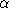## CGAL::Union_find<T,A>

### Definition

An instance P of the data type Union_find<T,A> is a partition of values of type T into disjoint sets. The template parameter A has to be a model of the allocator concept as defined in the C++ standard. It has a default argument CGAL_ALLOCATOR(T).

### Types

 Union_find::value_type values stored in items (equal to T). Union_find::handle handle to values. Union_find::iterator iterator over values.

There are also constant versions const_handle and const_iterator.

 Union_find::allocator allocator.

### Creation

 Union_find P; creates an instance P of type Union_find and initializes it to the empty partition.

### Operations

allocator P.get_allocator () the allocator of P.

std::size_t P.number_of_sets () returns the number of disjoint sets of P.

std::size_t P.size () returns the number of values of P.

std::size_t P.bytes () returns the memory consumed by P.

std::size_t P.size ( const_handle p) returns the size of the set containing p.

void P.clear () reinitializes P to an empty partition.

handle P.make_set ( T x) creates a new singleton set containing x and returns a handle to it.

handle P.push_back ( T x) same as make_set(x).

template <class Forward_iterator>
void P.insert ( Forward_iterator first, Forward_iterator beyond)
insert the range of values referenced by [first,beyond).
 Requirement: value type of Forward_iterator is T.

handle P.find ( handle p)
const_handle P.find ( const_handle p) returns a canonical handle of the set that contains p, i.e., P.same_set(p,q) iff P.find(p) and P.find(q) return the same handle.
 Precondition: p is a handle in P.

void P.unify_sets ( handle p, handle q)
unites the sets of partition P containing p and q.
 Precondition: p and q are in P.

bool P.same_set ( const_handle p, const_handle q)
returns true iff p and q belong to the same set of P.
 Precondition: p and q are in P.

iterator P.begin () returns an iterator pointing to the first value of P.

iterator P.end () returns an iterator pointing beyond the last value of P.

### Implementation

Union_find<T,A> is implemented with union by rank and path compression. The running time for m set operations on n elements is O(n(m,n)) where(m,n) is the extremely slow growing inverse of Ackermann's function.